JMSLTM Numerical Library 7.2.0
com.imsl.math

Class CsInterpolate

• All Implemented Interfaces:
Serializable, Cloneable

public class CsInterpolate
extends Spline
Extension of the Spline class to interpolate data points.

CsInterpolate computes a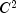cubic spline interpolant to a set of data points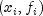for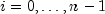. The breakpoints of the spline are the abscissas. Endpoint conditions can be automatically determined by the program, or explicitly specified by using the appropriate constructor. Constructors are provided that allow setting specific values for first or second derivative values at the endpoints, or for specifying conditions that correspond to the "not-a-knot" condition (see de Boor 1978).

The "not-a-knot" conditions require that the third derivative of the spline be continuous at the second and next-to-last breakpoint. If n is 2 or 3, then the linear or quadratic interpolating polynomial is computed, respectively.

If the data points arise from the values of a smooth, say,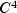function f, i.e.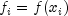, then the error will behave in a predictable fashion. Letbe the breakpoint vector for the above spline interpolant. Then, the maximum absolute error satisfies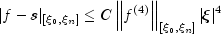where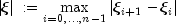For more details, see de Boor (1978, pages 55-56).

Example, Serialized Form
• Constructor Detail

• CsInterpolate

public CsInterpolate(double[] xData,
double[] yData)
Constructs a cubic spline that interpolates the given data points. The interpolant satisfies the "not-a-knot" condition.
Parameters:
xData - A double array containing the x-coordinates of the data. Values must be distinct.
yData - A double array containing the y-coordinates of the data. The arrays xData and yData must have the same length.
• CsInterpolate

public CsInterpolate(double[] xData,
double[] yData,
int typeLeft,
double valueLeft,
int typeRight,
double valueRight)
Constructs a cubic spline that interpolates the given data points with specified derivative endpoint conditions.
Parameters:
xData - A double array containing the x-coordinates of the data. Values must be distinct.
yData - A double array containing the y-coordinates of the data. The arrays xData and yData must have the same length.
typeLeft - An int denoting the type of condition at the left endpoint. This can be NOT_A_KNOT, FIRST_DERIVATIVE or SECOND_DERIVATIVE.
valueLeft - A double value at the left endpoint. If typeLeft is NOT_A_KNOT this is ignored, Otherwise, it is the value of the specified derivative.
typeRight - An int denoting the type of condition at the right endpoint. This can be NOT_A_KNOT, FIRST_DERIVATIVE or SECOND_DERIVATIVE.
valueRight - A double value at the right endpoint.
JMSLTM Numerical Library 7.2.0